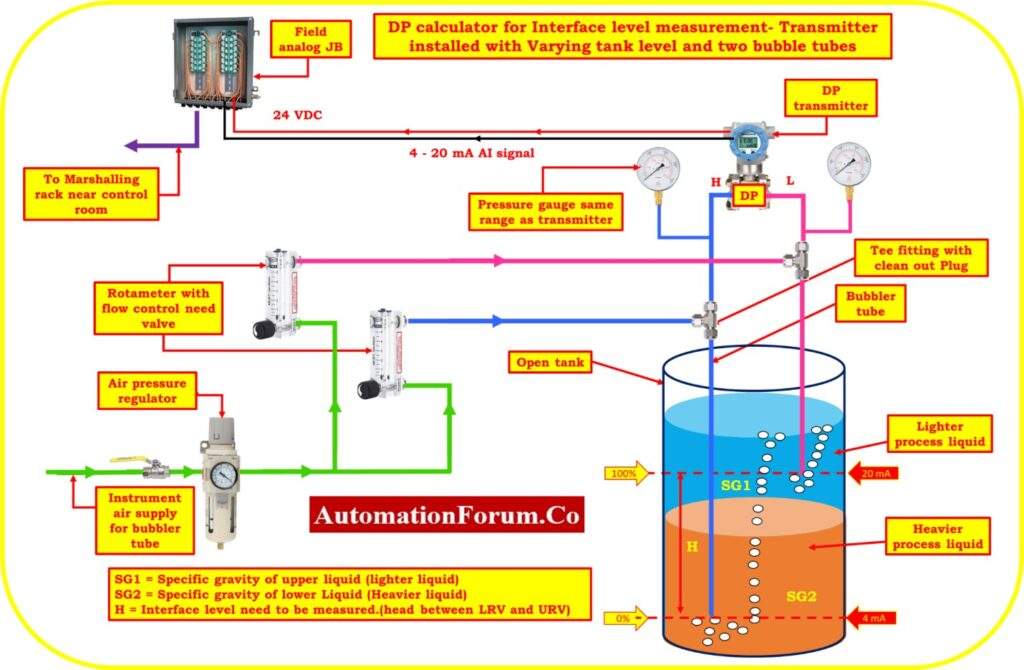# DP calculator for Interface level measurement – Transmitter installed with Varying Tank Level and two Bubble TubesA differential pressure (DP) transmitter can be used to measure the interface level in a tank with two bubble tubes. The transmitter measures the difference in pressure between the liquid levels in each of the bubble tubes, which is proportional to the interface level.

To calculate the interface level using the DP transmitter, several factors need to be considered, such as the distance between the bubble tubes and the bottom of the tank, the specific gravity of each liquid layer, and the total liquid height in the tank. The DP measurement is then converted into an interface level using a formula that takes into account the specific gravity difference between the two liquids.

Ultimately, the DP calculator for interface level measurement with varying tank level and two bubble tubes provides a reliable method for monitoring the interface level in a tank with different liquids.

LRV = Lower-range value (a measurement that’s output is 4 mA).

URV = Upper-range value (a measurement with a 20 mA output).

SG = A liquid’s specific gravity (relative density). Water has a specific gravity of 1.00 in both traditional and SI systems.

HW= Equivalent Headlevel pressure of Water

SG1 = Specific gravity of upper liquid (lighter liquid)

SG2 = Specific gravity of lower Liquid (Heavier liquid)

H = Interface level need to be measured.(Head between LRV and URV)

Calibrated Range of the transmitter = LRV to URV

## Formula for Span value calculation

Span value of the transmitter =   (H)*(SG2 – SG1)

## Formula for LRV estimation

Equivalent Head level pressure of Water (HW) at Lower range value

HW at LRV = (H*SG1) = Suppression

## Formula for URV estimation

Equivalent Head level pressure of Water (HW) at Higher range value

HW at URV = (H*SG2)

## DP calculator

This below calculator used to calculate the Span value, LRV and URV of the transmitter for calibration in interface level measurement